Refer to our Texas Go Math Grade 6 Answer Key Pdf to score good marks in the exams. Test yourself by practicing the problems from Texas Go Math Grade 6 Module 3 Quiz Answer Key.

3.1 Multiplying Fractions

Multiply.

Question 1.
$$\frac{4}{5} \times \frac{3}{4}$$
First we need to write the problem as a single fraction.
After that, we need to multiply numerators and denominators and then simplify by dividing by the GCF. Here, the GCF of 12 and 20 is 4.
Finally, we will write the answer in the simplest form. So, we have the following:So, the product is $$\frac{3}{5}$$

$$\frac{5}{7} \times \frac{9}{10}$$
First, we need to write the problem as a single fraction.
After that, we need to multiply numerators and denominators and then simplify by dividing by the GCF. Here, the GCF of 45 and 70 is 5.
Finally, we will write the answer in the simplest form. So, we have the following:So, the product is $$\frac{9}{14}$$.

Question 3.
Fred had 264 books in his personal library. He donated $$\frac{2}{11}$$ of these books to the public library. How many books did he donate?
In order to find how many books Fred donated, we have to multiply 264 by $$\frac{2}{11}$$ so, we have the following: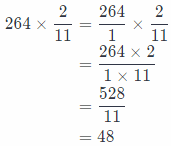The conclusion is that Fred donated 48 books to the public library.

3.2 Multiplying Mixed Numbers

Multiply.

Question 4.
$$\frac{3}{8} \times 2 \frac{1}{2}$$
First we need to rewrite the mixed number as a fraction greater than 1. After that we have to simplify before multiplying using the GCF and rewrite the fraction. Finally, multiply numerators and denominators and write the result in the simplest form.
So, we have the following: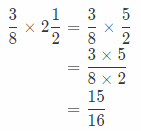The result is $$\frac{15}{16}$$.

$$3 \frac{3}{5} \times \frac{5}{6}$$
First, we need to rewrite the mixed number as a fraction greater than 1. After that, we have to simplify before multiplying using the GCF and rewrite the fraction. Finally, multiply numerators and denominators and write the result in the simplest form.
So, we have the following:The result is 3.

Question 6.
Jamal and Dorothy were hiking and had a choice between two trails. One was 5$$\frac{1}{3}$$ miles long, and the other was 1$$\frac{3}{4}$$ times as long. How long was the longer trail?
In order to calculate how long was the longer trail, we have to multiply 5$$\frac{1}{3}$$ and 1$$\frac{3}{4}$$.
We have to rewrite each mixed number as a fraction greater than 1.
Then multiply numerators and denominators, but before that we need to simplify using the GCF and rewrite the fraction.
At the end, we will write the result in the simplest form.
We have the following:So, the longer trail was 9$$\frac{1}{3}$$ miles long.

3.3 Dividing Fractions

Divide.

Question 7.
$$\frac{7}{8} \div \frac{3}{4}$$
First, we will rewrite division as multiplication, using the reciprocal of the division. After that we will multiply the numerators and denominators and finally write the answer in the simplest form. So, we have the following:The result is $$\frac{4}{3}$$.

$$\frac{4}{5} \div \frac{6}{7}$$
First, we will rewrite division as multiplication, using the reciprocal of the division. After that, we will multiply the numerators and denominators and finally write the answer in the simplest form. So, we have the following:The result is $$\frac{14}{15}$$.

Question 9.
$$\frac{1}{3} \div \frac{7}{9}$$
First, we will rewrite division as multiplication, using the reciprocal of the division. After that we will multiply the numerators and denominators and finally write the answer in the simplest form. So, we have the following:The result is $$\frac{3}{7}$$.

Question 10.
$$\frac{1}{3} \div \frac{5}{8}$$
First, we will rewrite division as multiplication, using the reciprocal of the division. After that we will multiply the numerators and denominators and finally write the answer in the simplest form. So, we have the following: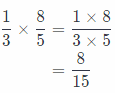The result is $$\frac{8}{15}$$.

3.4 Dividing Mixed Numbers

Divide.

Question 11.
$$3 \frac{1}{3} \div \frac{2}{3}$$
First we need to rewrite the mixed numbers as a fractions grater than 1.
Then rewrite the problem as multiplication using the reciprocal of the second fraction. After that, we will simplify using the GCF and multiply the numerators and denominators. Finally, we will write the result as a mixed number if it is possible. So, we have the following: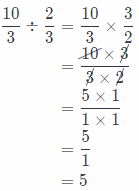The result is 5.

$$1 \frac{7}{8} \div 2 \frac{2}{5}$$
First, we need to rewrite the mixed numbers as fractions grater than 1.
Then rewrite the problem as multiplication using the reciprocal of the second fraction. After that, we will simplify using the GCF and multiply the numerators and denominators. Finally, we will write the result as a mixed number if it is possible. So, we have the following:The result is $$\frac{25}{32}$$

Question 13.
$$4 \frac{1}{4} \div 4 \frac{1}{2}$$
First we need to rewrite the mixed numbers as fractions grater than 1.
Then rewrite the problem as multiplication using the reciprocal of the second fraction. After that, we will simplify using the GCF and multiply the numerators and denominators. Finally, we will write the result as a mixed number if it is possible. So, we have the following: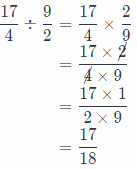The result is $$\frac{17}{18}$$

Question 14.
$$8 \frac{1}{3} \div 4 \frac{2}{7}$$
First we need to rewrite the mixed numbers as a fractions grater than 1.
Then rewrite the problem as multiplication using the reciprocal of the second fraction. After that, we will simplify using the GCF and multiply the numerators and denominators. Finally, we will write the result as a mixed number if it is possible. So, we have the following: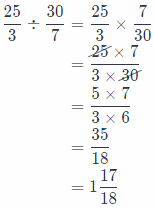The result is $$\frac{35}{18}$$ = 1$$\frac{17}{18}$$.

Essential Question

Describe a real-world situation that is modeled by multiplying two fractions or mixed numbers.
For, example, a real-world situation that would be appropriate to be modeled by multiplying two fractions or mixed numbers is the following.
Jane has 150$$\frac{1}{2}$$ grams of chocolate and she wants to split it into three friends.
How much chocolate will each friend get?

Jane has 150$$\frac{1}{2}$$ grams of chocolate and she wants to split it into three friends.
How much chocolate will each friend get?

Texas Go Math Grade 6 Module 3 Mixed Review Texas Test Prep Answer Key

Texas Test Prep

Question 1.
Which of the following statements is correct?
(A) The product of $$\frac{3}{4}$$ and $$\frac{7}{8}$$ is less than $$\frac{7}{8}$$.
(B) The product of 1$$\frac{1}{3}$$ and $$\frac{9}{10}$$ is less than $$\frac{9}{10}$$.
(C) The product of $$\frac{3}{4}$$ and $$\frac{7}{8}$$ is greater than $$\frac{7}{8}$$.
(D) The product of $$\frac{7}{8}$$ and $$\frac{9}{10}$$ is greater than $$\frac{9}{10}$$.
(A) The product of $$\frac{3}{4}$$ and $$\frac{7}{8}$$ is less than $$\frac{7}{8}$$.

Explaination:
The correct answer is A. Really:
$$\frac{3}{4} \times \frac{7}{8}$$ = $$\frac{3 \times 7}{4 \times 8}$$
= $$\frac{21}{32}$$

And
$$\frac{7}{8}$$ = $$\frac{7 \times 4}{8 \times 4}$$
= $$\frac{28}{32}$$

We can notice that:
$$\frac{3}{4} \times \frac{7}{8}=\frac{21}{32}<\frac{28}{32}=\frac{7}{8}$$

Question 2.
Which shows the GCF of 18 and 24 with $$\frac{18}{24}$$ in simplest form?
(A) GCF: 3; $$\frac{3}{4}$$
(B) GCF: 3; $$\frac{6}{8}$$
(C) GCF: 6; $$\frac{3}{4}$$
(D) GCF: 6; $$\frac{6}{8}$$
(C) GCF: 6; $$\frac{3}{4}$$

Explaination:
GCF for 48 and 24 is 6, so, we have the following form of this fraction:
$$\frac{18}{24}=\frac{18 \div 6}{24 \div 6}=\frac{3}{4}$$
Conclusion is that correct answer is C.

A jar contains 133 pennies. A bigger jar contains 1$$\frac{2}{7}$$ times as many pennies. What is the value of the pennies in the bigger jar?
(A) $1.49 (B)$ 1.52
(C) $1.68 (D)$ 1.71
(D) \$ 1.71

Explaination:
The value of pennies in the bigger jar is product of 133 and 1$$\frac{2}{7}$$, so we have the following: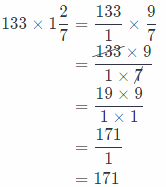So, the value of pennies in the bigger jar is 171.
Conclusion is that correct answer is D.

Question 4.
Which of these is the same as $$\frac{3}{5}$$ ÷ $$\frac{4}{7}$$?
(A) $$\frac{3}{5} \div \frac{7}{4}$$
(B) $$\frac{4}{7} \div \frac{3}{5}$$
(C) $$\frac{3}{5} \times \frac{4}{7}$$
(D) $$\frac{3}{5} \times \frac{7}{4}$$
(D) $$\frac{3}{5} \times \frac{7}{4}$$

Explaination:
The first step at dividing two factors is to rewrite the problem as multiplication
Using the reciprocal of the second fraction.
Here, reciprocal of $$\frac{4}{7}$$ is $$\frac{7}{4}$$ and equivalent is expression $$\frac{3}{5} \times \frac{7}{4}$$
Conclusion is that correct answer is D.

Question 5.
What is the reciprocal of 3$$\frac{3}{7}$$?
(A) $$\frac{7}{24}$$
(B) $$\frac{3}{7}$$
(C) $$\frac{7}{3}$$
(D) $$\frac{24}{7}$$
(A) $$\frac{7}{24}$$

Explaination:
First we will find mixed number as a fraction greater than 1:
3$$\frac{3}{7}$$ = $$\frac{24}{7}$$
The reciprocal of $$\frac{24}{7}$$ is $$\frac{7}{24}$$. So, conclusion is that correct answer is A.

Question 6.
A rectangular patio has a length of 12$$\frac{1}{2}$$ feet and an area of 103$$\frac{1}{8}$$ square feet. What is the width of the patio?
(A) 4$$\frac{1}{8}$$ feet
(B) 8$$\frac{1}{4}$$ feet
(C) 16$$\frac{1}{2}$$ feet
(D) 33 feet
(B) 8$$\frac{1}{4}$$ feet

Explaination:
The width of the patio we will dividing the area of patio, 103$$\frac{1}{8}$$, by its length, 12$$\frac{1}{2}$$
So, we have the following:So, the width of the patio is 8$$\frac{1}{4}$$ feet. The correct answer is B.

Gridded Response

Jodi is cutting out pieces of paper that measure 8$$\frac{1}{2}$$ inches by 11 inches from a large sheet that has an area of 1,000 square inches. What is the area of each piece of paper that Jodi is cutting out written as a decimal?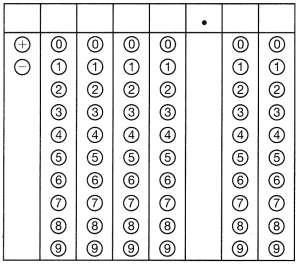The area of each piece of paper is product of 8$$\frac{1}{2}$$ and 11:
8$$\frac{1}{2}$$ × 11 = $$\frac{17}{2} \times \frac{11}{1}$$
= $$\frac{17 \times 11}{2}$$
= $$\frac{187}{2}$$